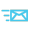•0086-0577-62698923
•sales@huimultd.com
•No.266 Liujiang Road, Wenzhou, Zhejiang, China

location : Home > Terminology Library >

# Power2019-07-11

Power refers to the amount of work done by an object in a unit of time. That is, the power is a physical quantity that describes the speed of work, and can be divided into electric power and power.

The electric power is the physical quantity indicating the current work speed. The power of a electronic component is equal to the power consumed by it in 1 second (t, unit is s) (W, the unit is J), that is, P=W/t.

The magnitude of the electric power is also equal to the product of the voltage across the conductor and the current through the conductor: P = U*I.

For a purely resistive circuit, the calculation formula of electric power can also be expressed as: P=I^2*R and P=U^2 /R.